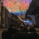4819 views
A quadratic regression is the process of finding the equation that best fits a set of data.This form of regression is mainly used for smoothing data shaped like a parabola.

Because we can use short/midterm/longterm periods we can say that we use a Quadratic Least Squares Moving Average or a Moving Quadratic Regression.

Like the Linear Regression ( LSMA ) a Quadratic regression attempt to minimize the sum of squares (sum of the squared difference between a set of data and an estimator), this is why
those kinds of filters have low lag.

Here the difference between a Least Squared Moving Average (green) and a Quadratic Regression (red) of both period 500

Here it look like the Quadratic Regression have a best fit than the LSMA
Open-source script

In true TradingView spirit, the author of this script has published it open-source, so traders can understand and verify it. Cheers to the author! You may use it for free, but reuse of this code in a publication is governed by House Rules. You can favorite it to use it on a chart.

Want to use this script on a chart?
Patreon: https://www.patreon.com/alexgrover

You can also check out some of the indicators I made for luxalgo : https://www.tradingview.com/u/LuxAlgo/#published-scriptsHey @alexgrover, have you ever tried implementing any more sophisticated forms of regression? I've been interested in seeing what ridge regression or lasso regression does on the charts.

Regards